Technical Information Site of Power Supply Design

2020.07.08 Si Power Device

# MOSFET Thermal Resistance and Power Dissipation: Packages Capable of Back-Surface Heat Dissipation

Si Transistors

When designing a circuit, thermal calculations are indispensable, and particularly where power devices that handle large amounts of power are concerned, they are extremely important, not only with respect to operation lifetime but from the standpoint of safety as well. This and the following article are devoted to an explanation of power dissipation and thermal resistance of MOSFETs. In this article, we explain packages that are capable of heat dissipation from the back surface; in the next article we discuss packages not capable of such back-surface heat dissipation.

Thermal Resistance of Packages Capable of Back-Surface Heat Dissipation

Below are presented terms and definitions relating to the thermal resistance of packages that can dissipate heat from the back surface, as is the case with packages onto which heat sinks can be installed such as the TO-220FM and the TO-247, and packages a back-surface pin of which can be mounted onto a circuit board, such as the TO-252 and the TO-263.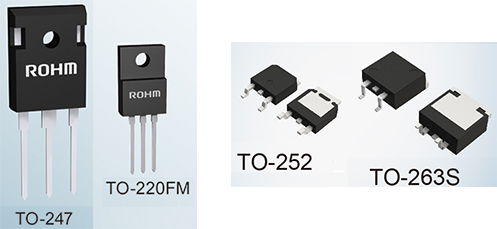• TA： Ambient (atmosphere) temperature
• TJ： Junction temperature
• TC： Package back-surface temperature
• TT： Package marking-surface temperature
• RthJAJA): Thermal resistance between junction and ambient (atmosphere)
• RthJCJC): Thermal resistance between junction and package back surface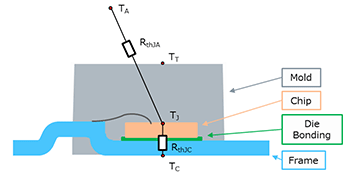The basic structure of a package able to dissipate heat from the back surface consists of a lead frame ("Frame" in the figure), die bonding between the chip and the lead frame, the MOSFET chip ("Chip"), and the resin package ("Mold"). The "junction" in the explanation of symbols refer to the PN junction, or put more simply, the chip.

The table below is an example of the absolute maximum ratings and thermal resistances appearing on a data sheet for an Nch MOSFET in a TO-247 package. Here TJ is stipulated as an absolute maximum rating, and so in thermal calculations the need to keep the absolute maximum rating TJ (also sometimes written TJMAX) from being exceeded is the ultimate requirement. Here RthJA, which is of fundamental importance for the thermal resistance, and RthJC, which is necessary for thermal calculations when the back surface of the package is mounted on the board or when a heat sink is mounted in close contact, are shown.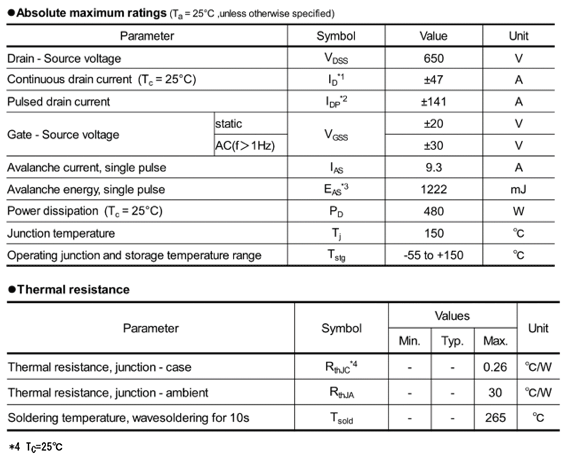Relationship Between Thermal Resistance and Power Dissipation

In the above absolute maximum ratings table, the power dissipation PD is indicated. PD and the thermal resistance RthJC are related by the following equation.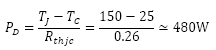For TJ the absolute maximum rating of 150°C is substituted, and for TC, 25°C, which is the condition for the power dissipation, is used. For RthJC, the maximum value of 0.26°C/W is substituted. As indicated in note *4, RthJC is the value at TC=25°C, and so matches the condition stipulated for PD. The calculation result is approximately 480 W,　and is the value of PD appearing in the table.

To make this equation a little easier to understand, the difference between TC and the absolute maximum rating TJ is the allowed value for heat generated by the chip itself. For the above conditions this is 150 – 25 = 125°C, and the chip is allowed to generate heat up to 125°C. Heat generation is the thermal resistance times the power consumption, and so by dividing the allowable heat generation by the thermal resistance, the power consumption that can be allowed, that is, the power dissipation is obtained.

Here there is a matter that must be understood. The provided value of RthJC has the condition that TC = 25°C. Put another way, if TC is not 25°C, RthJC is not 0.26°C/W. When considering actual conditions of use, the condition that TC = 25°C is hardly ever satisfied, and so the RthJC presented on the data sheet as an example cannot be used in thermal calculations for the actual conditions of use. It may seem contradictory for a data sheet to present data that cannot actually be used, but in many cases, specific conditions are required in order to stipulate specification values, and it is understood that specified values on a data sheet, whether for thermal resistance or anything else, are values stated for particular conditions.

Hence in order to use RthJC to determine the power dissipation under actual conditions of use, the RthJC under those conditions must be measured and ascertained. However, this in turn requires corresponding measurement instruments, environment preparations, and the like.

As one other method, RthJA can be used. The relationship between TJ and RthJA can be expressed by the following equation.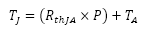P is the power loss (power consumption). The quantity within parentheses corresponds to the heat generated by the chip itself; this plus the ambient temperature TA is equal to TJ. It should be considered that by using RthJA, TA can be substituted for TC in the previous equation. Through this calculation, the power loss P or TA is adjusted such that TJ does not exceed the absolute maximum rating of 150°C.

In order to use this equation in calculations, the ambient temperature, the power loss of the MOSFET, and RthJA must be determined. The ambient temperature and the power loss can be ascertained relatively easily. The RthJA for this kind of package varies depending on the soldered pad area of the package back surface for mounting on the board, the thickness of the copper foil, and the material and number of layers of the board. In some cases it is possible to obtain the RthJA for mounting under standard board conditions.

#### Key Points:

・In thermal calculations, various thermal resistances and the power loss are used to determine TJ, to confirm that ultimately the absolute maximum rating for TJ is not exceeded.

・The power dissipation is obtained by calculating backward to the power loss for which TJ does not exceed the absolute maximum rating.

・Specified values, absolute maximum ratings and the like must be considered paying attention to the stipulated conditions.